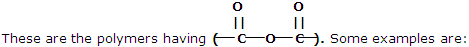solution and colligative properties

what is molarity of a solution of hcl which contains 49% by weight of solute and whose specific gravity is 1.41

#### Related Questions in Chemistry

• ##### Q :Unit of molality Select the right

Select the right answer of the question. The unit of molality is: (a) Mole per litre (b) Mole per kilogram (c) Per mole per litre (d) Mole litre

• ##### Q :What are condensation polymers? Give

These types of polymers are formed as a result of condensation reaction between monomer units. Some common examples are being discussed here:

1. Polyesters##### Q :Define Virial Equation The constant of

The constant of vander Waal's equation can be related to the coefficients of the virial equation.

Vander Waal's equation provides a good overall description of the real gas PVT behaviour. Now let us

• ##### Q :What do you mean by the term Organic

What do you mean by the term Organic Chemistry? Briefly define the term?

• ##### Q :Define Bond Energies - Bond Charges

Energy changes in some chemical reactions can be used to deduce the energies of chemical bonds.

Our understanding of the molecular basis of thermodynamic properties is extended when we ask why the enthalpy change for a reaction is what it is. We deduce,

• ##### Q :Vapour pressure of volatile substance

Provide solution of this question. According to Raoult's law the relative lowering of vapour pressure of a solution of volatile substance is equal to: (a) Mole fraction of the solvent (b) Mole fraction of the solute (c) Weight percentage of a solute (d) Weight perc

• ##### Q :Problem on Adiabatic expansion

Calculate the change in entropy for the system for each of the following cases. Explain the sign that you obtain by a physical argument

a) A gas undergoes a reversible, adiabatic expansion from an initial state at 500 K, 1 MPa, and

• ##### Q :Determining maximum Osmotic pressure

Which of the following would have the maximum osmotic pressure (assume that all salts are 90% dissociated):

(a) Decimolar aluminium sulphate (b) Decimolar barium chloride (c) Decimolar sodium sulphate (d) A solution obtained by mix

• ##### Q :Problem on physical and thermodynamic

The shells of marine organisms contain calcium carbonate CaCO3, largely in a crystalline form known as calcite. There is a second crystalline form of calcium carbonate known as aragonite. Physical and thermodynamic properties of calcite and aragonite at 298

• ##### Q :Laws of Chemical Combination Laws of

Laws of Chemical Combination- In order to understand the composition of the compounds, it is necessary to have a theory which accounts for both qualitative and quantitative observations during chem